Analysis WebNotes
arrow_back arrow_forward

# Class Contents

## Sets Which Are Both Open and Closed

We already saw when we studied open and closed sets in the real line that it's possible for a set to be both open and closed. In general, in any metric space, the whole space X, and the empty set are always both open and closed.

This means that being open or closed are not mutually exclusive alternatives. You could say that openness and closedness are opposite concepts, but the way in which they are opposites is expressed by Proposition 5.12. Because the whole space and the empty set are always open and closed, they are said to be trivial examples of sets which are both open and closed.

It is natural to wonder whether there are any non-trivial examples of sets which are both open and closed. The answer to this question turns out to involve some deep questions about the shape (or, more accurately, the "topology") of the metric space under consideration.

We shall begin to explore this question by looking at some examples of sets which are both open and closed.

Example:

In the metric space of the integers, with the usual Euclidean distance between points, every set is open, and so every set is also closed.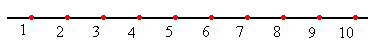Proof.

Example: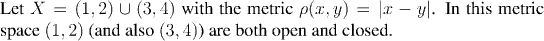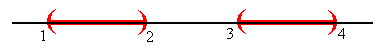Proof.

The set (1,2) can be viewed as a subset of both the metric space X of this last example, or as a subset of the real line. Clearly (1,2) is not closed as a subset of the real line, but it is closed as a subset of this metric space. The lesson of this, is that whether or not a set is open or closed can depend as much on what metric space it is contained in, as on the intrinsic properties of the set.

Our final example of a set which is both open and closed also serves to further illustrate this point: whether or not a set is open or closed depends crucially on what metric space it is in.

Example: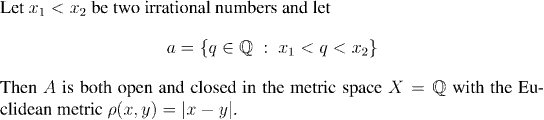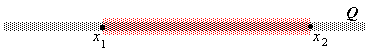Proof.

Note that as a subset of the real numbers, A is neither open nor closed.

Remark:

In all of these cases, when you look at the picture of the metric space in question the space "falls apart" naturally into a number of pieces. (Perhaps this isn't so obvious with the last example, bust you might think of the rational numbers as falling apart "like dust", into so many pieces that they're scattered everywhere.) This is a common feature of metric spaces which have a non-trivial open and closed set: they are all "disconnected", which is to say that they fall into two "separated" pieces. We'll come to a formal definition of "disconnected" in the next section. For the moment, let's look a little at the consequences for a metric space X of its having a non-trivial set which is both open and closed.

If A is a non-trivial open and closed subset of X, then A is neither X itself, nor the empty set. Thus, both A and its complement B are non-empty. We assumend that A was open, and since A is also closed, its complement B is open too.

Thus, we can conclude that when X has a non-trivial open and closed set,

X is the union of two disjoint, non-empty open sets
This is the property that we shall take to say that X is disconnected. It falls naturally into two parts; the two disjoint open sets that make it up.

The formal definition of disconnected is slightly more complicated than this, only because we want to be able to talk about subsets of X being disconnected, as well as just the whole space.